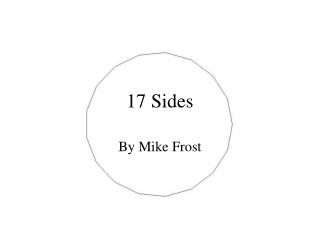DownloadDownload Presentation17 Sides

# 17 Sides

Télécharger la présentation## 17 Sides

- - - - - - - - - - - - - - - - - - - - - - - - - - - E N D - - - - - - - - - - - - - - - - - - - - - - - - - - -
##### Presentation Transcript

1. 17 Sides By Mike Frost

2. What Can You Construct with Straight Edge and Compass? Lengths can be multiplied

3. Lengths can be halved by Dropping a Perpendicular

4. Angles can be Halved

5. Square Roots can be taken But you can’t: Trisect an Angle or take Cube Roots

6. Constructing the Pentagon

7. To construct a Polygon with a prime number of sides n, n must be a Fermat Prime, of the form: F0 = 3 Five known Fermat Primes N=0,1,2,3,4 F1 = 5 F2 = 17 F3 = 257 F4 = 65, 537 Carl Friedrich Gauss

8. “Dr Euler’s Fabulous Formula” – by Paul Nahin

9. Cos (2 π / 17) ... Cos (2 π / 3) = -0.5 Cos (2 π / 5) = (-1 + sqrt(5) ) / 4 Cos (2 π / 257) ?

10. ...and Cos (2π/65,537) ??

11. The Biggest ConstructibleOdd-Numbered Polygon3 . 5 . 17 . 257 . 65537= 4 , 294 , 967 , 295 Sides

12. Constructing the 17-Gon